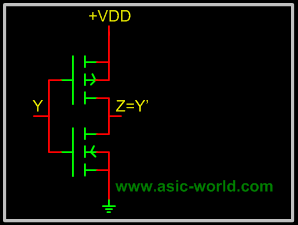Digital Logic Gates Part-VI Jan-4-2006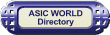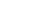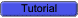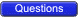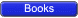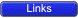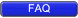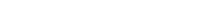Integrated Injection LogicTODOEmitter coupled logic Emitter coupled logic (ECL) is a non saturated logic. Which means that transistors are prevented from going into deep saturation, thus eliminating storage delays. Preventing the transistors from going into saturation is accomplished by using logic levels whose values are so close to each other that a transistor is not driven into saturation when its input switches from low to high. In other words, the transistor is switched on, but not completely on. This logic family is faster then TTL.Voltage level for high is -0.9 Volts and for low is -1.7V, Thus biggest problem with ECL is poor noise margin.A typical ECL OR gate is shown below. When any one the inputs is HIGH (-0.9v), Then transistor to which this input is connected will conduct, and hence will make the Q3 off, which in turn will make Q4 output HIGH.When both the inputs are LOW (-1.7v), Then transistor to which this input is connected will not conduct, and hence will make the Q3 on, which intern will make Q4 output LOW.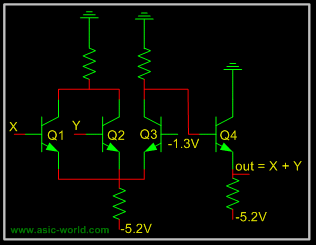Metal Oxide Semiconductor Logic MOS or Metal Oxide Semiconductor logic uses nmos and pmos to implement logic gates. One need to know operation of FET, MOS transistors to understand the operation of MOS logic circuits.The basic NMOS invertor is shown below, when input is LOW, NMOS transistor does not conduct, and thus output is HIGH. But when input is HIGH, NMOS transistor conducts and thus output is LOW.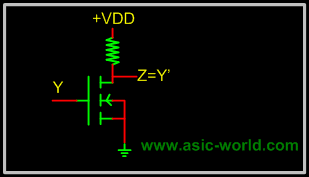Normally it is difficult to fabricate resistors inside the chips, so resistor is replaced with NMOS gate as shown below. This new NMOS transistor acts as resistor.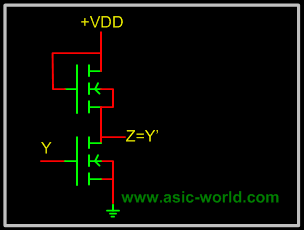Complimentary Metal Oxide Semiconductor Logic CMOS or Complimentary Metal Oxide Semiconductor logic is built using both NMOS and PMOS. Below is the basic CMOS inverter circuit, which follows following rules. NMOS conducts when its input is HIGH. PMOS conducts when its input is LOW. So when input is HIGH, NMOS conducts, and thus output is LOW, when input is LOW PMOS conducts and thus output is HIGH.